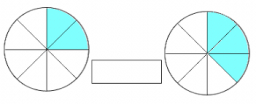# Why is

Why is three divided by one-fifth different from one-fifth divided by three?

a =  15
b =  0.0667

### Step-by-step explanation:Did you find an error or inaccuracy? Feel free to write us. Thank you!

Tips for related online calculators
Need help calculating sum, simplifying, or multiplying fractions? Try our fraction calculator.